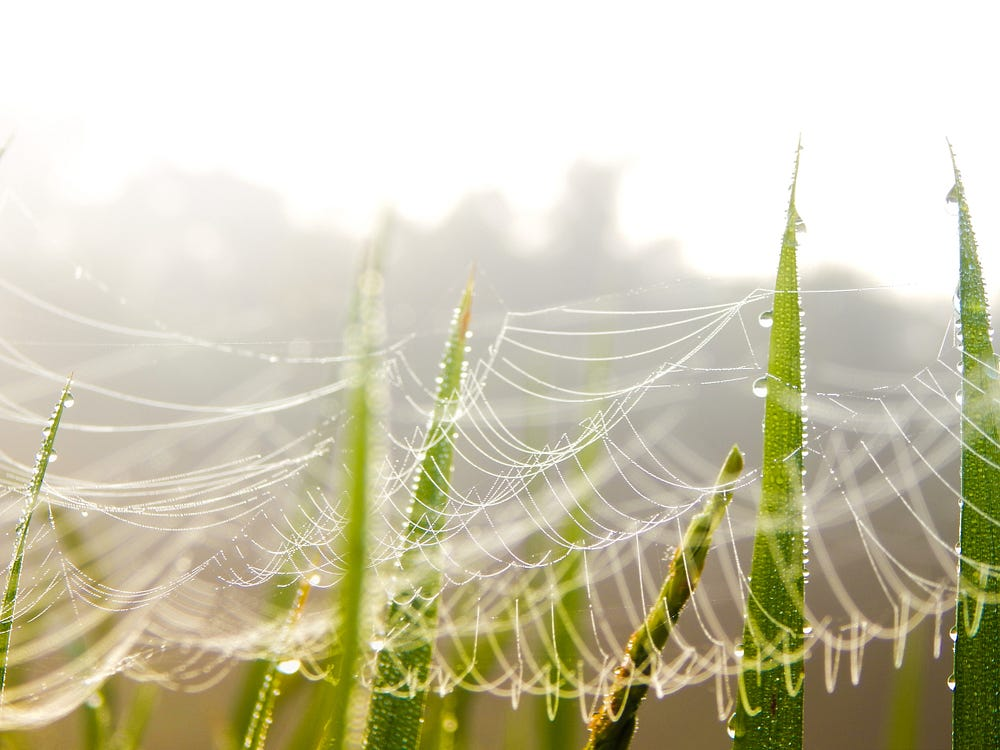# Elixir and Reinforcement Learning — IV

Source: Deep Learning on Medium## Let’s use the Neural Network

With the network code created, let’s make some changes to get our car to start using the network and no more keyboard commands.

Remember our `lib/scenes/environment.ex` file where we started the car, environment, etc? We need to call the neural network inside it now.

`# lib/scenes/environment.exdefmodule AutonomousCar.Scene.Environment do use Scenic.Scene require Logger alias Scenic.Graph alias Scenic.ViewPort alias AutonomousCar.Math.Vector2 alias AutonomousCar.Objects.Car alias AutonomousCar.NeuralNetwork.Network import Scenic.Primitives def init(_arg, opts) do ...(more)...`

Within the `init/2` method, where we store the `state`, we need to add the neural network pid, the car’s last position coordinates and the sensor signals. And we can’t forget to start the neural network `Network.start_link/1`. To keep up with our source of inspiration, which was the course of Udemy commented on Episode 1, I will initialize the network with 5 inputs, 30 hidden layer neurons and 3 output neurons.

`# lib/scenes/environment.exdef init(_arg, opts) do  ... # start neural network {:ok, neural_network_pid} = Network.start_link([5, 30, 3]) state = %{ viewport: viewport, viewport_width: viewport_width, viewport_height: viewport_height, graph: graph, frame_count: 0,neural_network_pid: neural_network_pid, objects: %{ car: %{ dimension: %{ width: 20, height: 10}, coords: {pos_x, pos_y},last_coords: {pos_x, pos_y}, velocity: {1, 0}, angle: 0,signal: %{ left: 0, center: 0, right: 0 }, sensor: %{ left: {0, 0}, center: {0, 0}, right: {0, 0} } }, goal: %{coords: {20,20}} } } ...`

We also need to modify the `handle_info/2` method to capture the current car data, the latest coordinates, send it to the neural network and position the car according to the objective.

`# lib/scenes/environment.ex...def handle_info(:frame, %{frame_count: frame_count} = state) do# Current car position {car_x, car_y} = state.objects.car.coords # Last car position {car_last_x, car_last_y} = state.objects.car.last_coords # Current goal position {goal_x, goal_y} = state.objects.goal.coords # Vector1 - Current position minus previous position vector1 = Scenic.Math.Vector2.sub({car_x, car_y}, {car_last_x, car_last_y}) # Vector2 - Current position goal minus previous position car vector2 = Scenic.Math.Vector2.sub({goal_x, goal_y}, {car_last_x, car_last_y}) # Normalized vector1 vector1_normalized = Scenic.Math.Vector2.normalize(vector1) # Normalized vector2 vector2_normalized = Scenic.Math.Vector2.normalize(vector2) # Orientation orientation = Scenic.Math.Vector2.dot(vector1_normalized, vector2_normalized) # Radius Orientation orientation_rad = Math.acos(orientation) # Grad orientation orientation_grad = (180 / :math.pi) * orientation_rad # Signals signal_sensor_1 = 0 signal_sensor_2 = 0 signal_sensor_3 = 0 # Deep Learning action = state.neural_network_pid |> Network.get() |> Network.predict([0, 0, 0, orientation, -orientation]) state = state |> Car.update_rotation(action) new_state = if rem(frame_count, 4) == 0 do state |> Car.move else state end graph = state.graph |> draw_objects(state.objects) {:noreply, new_state, push: graph}end ...`

The `handle_input/3` can be deleted.

`# lib/scenes/environment.ex...## Deleteddef handle_input({:key, {"left", :press, _}}, _context, state) do {:noreply, Car.update_rotation(state, 1)}enddef handle_input({:key, {"right", :press, _}}, _context, state) do {:noreply, Car.update_rotation(state, 2)}enddef handle_input(_input, _context, state), do: {:noreply, state} ...`